• 优化货架商品摆放，或优化邮寄商品目录的内容
• 交叉销售和捆绑销售
• 异常识别等

# 关于交易数据的表述形式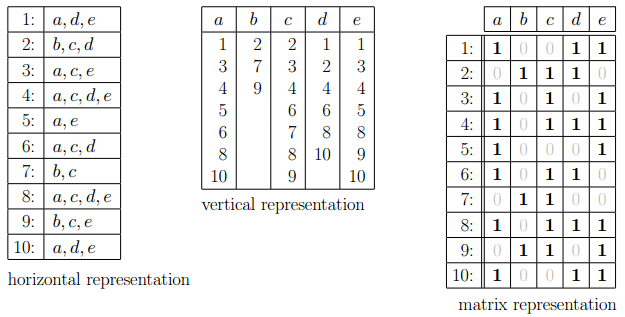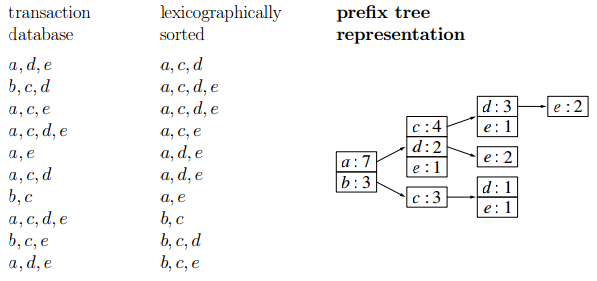• 排序表述（lexicographically sorted）
• 前缀树表述（prefix tree）

# Apriori 算法

Apriori算法是一种最有影响的挖掘 0-1 布尔关联规则频繁项集的算法。这种算法利用了频繁项集性质的先验知识（因此叫做priori）。Apriori使用了自底向上的实现方式（如果集合 I 不是频繁项集，那么包含 I 的更大的集合也不可能是频繁项集），k - 1 项集用于探索 k 项集。首先，找出频繁 1 项集的集合($$L_1$$)，$$L_1$$用于找频繁 2 项集的集合 $$L_2$$，而 $$L_2$$ 用于找 $$L_3$$，如此下去，直到不能找到满足条件的频繁 k 项集。搜索每个 $$L_k$$ 需要一次全表数据库扫描。

item support
{1} 3
{2} 6
{3} 4
{4} 5

1项集的最低频数是3，我们姑且认为他们都是频繁的。因此我们找到1项集所有可能组合的pairs：

item support
{1,2} 3
{1,3} 1
{1,4} 2
{2,3} 3
{2,4} 4
{3,4} 3

item support
{1,2} 3
{2,3} 3
{2,4} 4
{3,4} 3

# FP growth

FP growth 算法包含两部分：存储的FP tree 和对应的FP 算法：

## FP-tree 的结构

2. 每个子树上包含如下信息：
• item 的名称（比如下图中I2, I3, I5等）
• 计数（support count）：到达这个节点的路径深度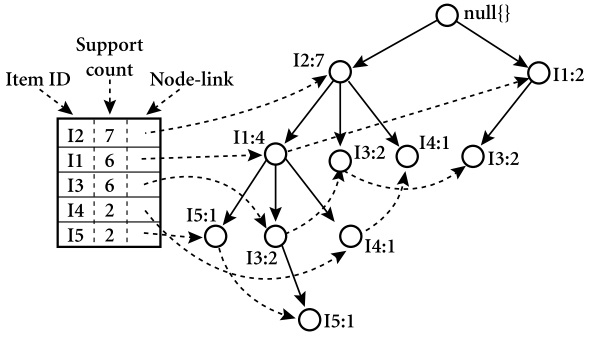## FP-tee 的算法

No. transactions Sort
2 BCE BEC
4 ABCE BEAC
5 ABCDE BEACD
6 BCD BCD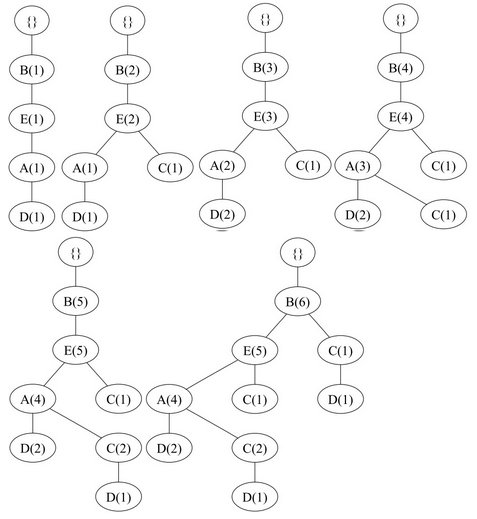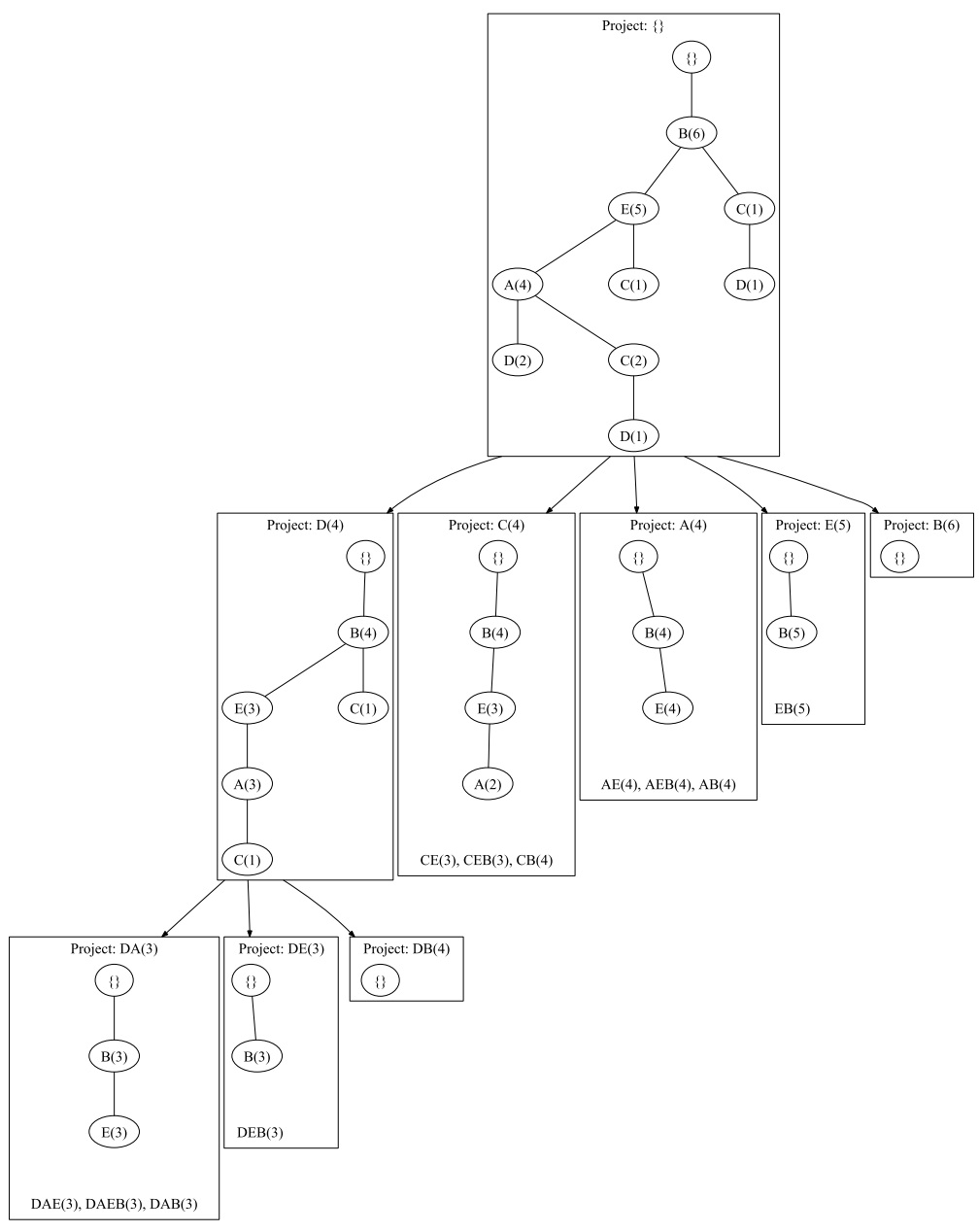• 对于 $$DA(3)$$ 的前缀路径是 $$Project:DA(3)$$ 的树形结构，因此这条线的最终结果是 $$DAE(3),DAEB(3),DAB(3)$$
• 对于 $$DE(3)$$ 的前缀路径是 $$Project:DE(3)$$ 的属性结构，最终结果是 $$DEB(3)$$
• 对于 $$DB(4)$$ 只有一个根，没有结果

## FP-tree 算法在R中的实现

• http://en.wikipedia.org/wiki/Association_rule_learning
• http://en.wikipedia.org/wiki/Apriori_algorithm
• http://www.borgelt.net//courses.html#fpm